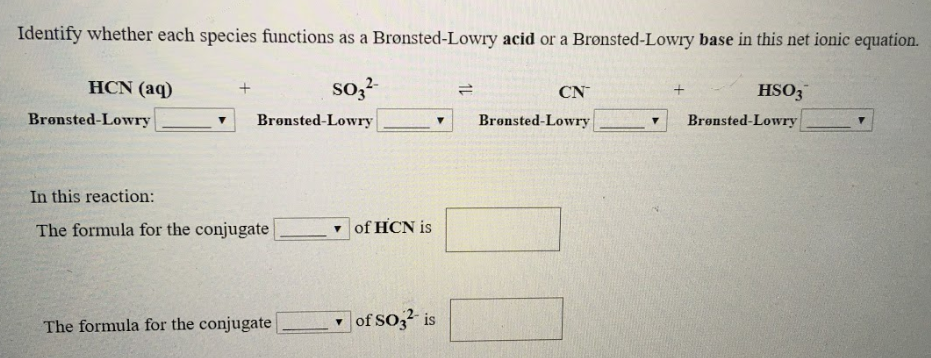# Identify whether each species functions as Bronsted-Lowry acid or a Bronsted-Lowry base in this net ionic equation. HCN(aq) + SO3^2- ⇌ CN^- + HSO3^- In this reaction: The formula for the conjugate ____ of HCN is ____ The formula for the conjugate _____ of SO3^2- is ____